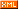# Nowpaper 十五英寸的世界

Rich Games Developer

 博客园  :: 首页  :: 新随笔  :: 联系 :: 订阅:: 管理<e:Group id="group_win" left="0" top="0" bottom="0" right="0" visible="false">
<e:Rect left="0" top="0" bottom="0" right="0" fillAlpha="0.53"/>
<e:Image source="Result_png" horizontalCenter="0" verticalCenter="260"/>
<e:Button id="btn_next" x="432" y="941">
<e:skinName>
<e:Skin states="up,down,disabled">
<e:Image width="100%" height="100%" source="ResultBtn_png" source.down="ResultBtn1_png"/>
<e:Label id="labelDisplay" horizontalCenter="0" verticalCenter="0"/>
</e:Skin>
</e:skinName>
</e:Button>
<e:Label id="lb_from" text="标签" x="113" y="700" textColor="0x000000" multiline="true" width="501" height="65"/>
<e:Label id="lb_explain" multiline="true" textColor="0x000000" text="标签" height="127" y="805" width="501" x="113"/>
</e:Group>

private group_win:eui.Group;//胜利界面的group控件
private btn_next:eui.Button;//下一个题目
private lb_explain:eui.Label;//解释
private lb_from:eui.Label;//来源

private onclick_next(){
//下一个题目
this.group_win.visible = false;
SceneLevels.Shared().OpenLevel(this.levelIndex + 1);
this.InitLevel(this.levelIndex + 1);

}
private showWin(){
this.group_win.visible = true;
var leveldata = LevelDataManager.Shared().GetLevel(this.levelIndex);
this.lb_from.text = leveldata.tip;
this.lb_explain.text = leveldata.content;
}

this.btn_next.addEventListener(egret.TouchEvent.TOUCH_TAP,this.onclick_next,this);

this.showWin();posted on 2016-02-26 13:29  nowpaper  阅读(3965)  评论(0编辑  收藏  举报